Algebra of Functions
 The graphing calculator can be used to investigate algebra of functions.

 Algebra of Functions: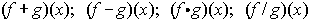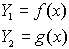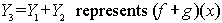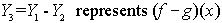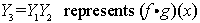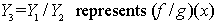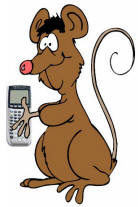* When entering the multiplication of functions, several notation entries are
possible: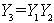OR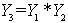BUT NOTas it is composition.

*
Remember when performing the division of functions, that zero denominators cause problems and will affect the look of the graph.

* Remember that you can find the Y-subscsripted variables under the VARS key: arrow right to Y-VARS, and choose 1. Function.

Example:

Given: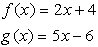a)  graph:b)  find: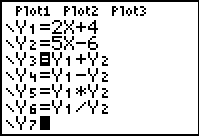Solution:
1.  Enter f (x) into Y1 and g(x) into Y2.
2.  Enter the expression for the operation you will be examining into Y3

(or into successive locations).
3.  Turn off the first two entries, as well as any other entries that you do not want graphed.
4.  Set an appropriate viewing window for the graph.
5.  Graph.  (this will be the answer to part a)

6.  While on the graphing screen, hit TRACE.  Simply enter the number you wish to find
(if that number is within the viewing window.)  Hit ENTER for the answer.
(this will be the answer to part b)
OR

From the home screen, enter Y3( 5 ), assuming your functions are entered in Y1 and
Y
2 with the operation expression in Y3.  Hit ENTER.
(this will be the answer to part b)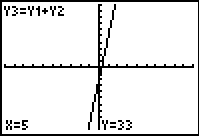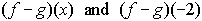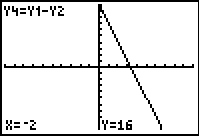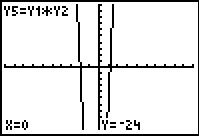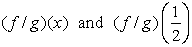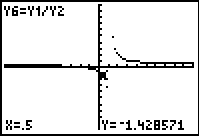Dot Mode was used here to avoid seeing the connected "heart beat" where the denominator equaled zero.